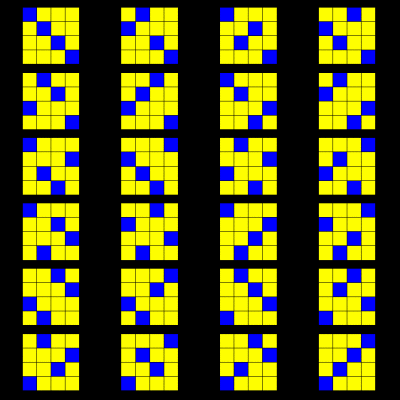M A T H 2 1 B
Mathematics Math21b Spring 2007
Linear Algebra and Differential Equations
Exhibit: determinant
 The determinant det(A) of a nxn matrix A is a number which tells for example, whether the matrix A is invertible or not. The most common mathematical definition is to define det(A) as the sum of all possible terms (-1)s(p) A1 p(1) A2 p(2) ...An p(n), where s(p) is the number of upcrossings, cases for which p(k) is smaller than p(l) for k smaller than l. The picture to the right shows all 24 permutations p in the case n=4. The number of upcrossings in the first permutation for example is 0, in the second 1, in the third 1, in the fourth 2. We rarely will compute the determinant with this definition but it has the advantage that all other methods for computing det(A) follow immediately. The book defines det(A) as an inductive Laplace expansion of the determinant which requires a lot of handwaving to justify facts. Justification is done on page 258, the most awful page of the entire book. When defining the determinant as a signed sum over all permutations, this page can be obmitted and nothing is lost because the Laplace expansion follows.